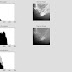clc;
clear;

%%%%%%%%%%%%%%%%%%%%%%%%%%%%%%%%%%%%%%%%%%%%%%%%%

hist = imhist(img);    %histogrm

numOfPixels = size(img,1)*size(img,2);

iMean = mean(mean(img));    % mean of input image

%%%%%%%%%%%%%%%%%%%%%%%%%%%%%%%%%%%%%%%%%%%%%%%%%%%%
%% histogram modification

g = zeros(256,1);

M = numOfPixels/256;

sai = 0.55;

for i = 1:256;
g(i) = (hist(i)+(sai*M))/(1+sai);
end

%%%%%%%%%%%%%%%%%%%%%%%%%%%%%%%%%%%%%%%%%%%%

prob = zeros(256,1);
cum = zeros(256,1);
output = zeros(256,1);

for i = 1:256;
prob(i) = g(i)/numOfPixels;
end

sum = 0;
for i = 1:256;
sum = sum+prob(i);
cum(i) = sum;
end

for i = 1:256;
output(i) = round(1+(256-1)*cum(i));
end

%%%%%%%%%%%%%%%%%%%%%%%%%%%%%%%%%%%%%%%%%%%%%%%%%%%%

HIm=uint8(zeros(size(img,1),size(img,2)));
for i=1:size(img,1)
for j=1:size(img,2)
HIm(i,j)=output(img(i,j)+1);
end
end

%%%%%%%%%%%%%%%%%%%%%%%%%%%%%%%%%%%%%%%%%%%%%%%%%%%
%% Homomorphic Filtering after modification

img_fft = (log(double(HIm) + 1 ));  % log

img_fft = fft2(img_fft);     % fourior transform

size_img = size(img_fft);
lpf = [1 1 1;1 1 1;1 1 1]/9;

lpf_fft = fft2 ( lpf , size_img ( 1 ) , size_img ( 2 ) );  %% applying filter
result_fft = img_fft .* lpf_fft;

result_img = ( ifft2(result_fft) );   %% inverse fourior transform

result_img = exp (result_img);
result_img = uint8(real ( result_img ));

%%%%%%%%%%%%%%%%%%%%%%%%%%%%%%%%%%%%%%%%%%%%%%%%%%

pMean = mean(mean(result_img));

result_img =  (iMean/pMean) .* result_img ;   %%normalize

subplot(3,2,1), imshow(img);
title('Original Image');

subplot(3,2,2), imshow(histeq(img));
title('HE Image');

title('CLAHE Image');

title('USM Image');

subplot(3,2,5), imshow(HIm);
title('Image Before Normalization');

subplot(3,2,6), imshow(result_img);
title('MH-FIL');

Mat Lab Code for Modified Histogram Based Contrast Enhancement Using Unsharp masking Filter For Medical ImagesReviewed by Suresh Bojja on 11/01/2015 06:51:00 AM Rating: 5

## Subjects & Labs

AC (6) ADS (2) ATMEL (6) AWP (5) C- Language (3) CAO (5) CDAC (1) CMC (6) CN (10) Control Sys (2) CVSM (2) DBMS (1) DC (5) DIP (17) DLD (10) DS (4) DSD&DICA (9) DSP (6) EDC (45) Embedded (7) EMWTL (6) ENERGY AUDIT (1) Engg.Chemistry (11) Faculty Labs (1) Fuzzy (3) II MID (1) LICA (1) Linux OS (1) Materials (1) MATLAB (27) MCU IDE 8051 (1) MEMS (4) MPMC (57) MTECH (6) MWE (2) OC (1) PDC (9) Projects (8) RADAR (1) Research (9) RVSP (3) SC (1) Softwares (1) SS (2) STLD (19) TCSS (1) TSSN (1) TV Engg (1) VHDL (4) VLSI (8) WCN (1) WSN (2)